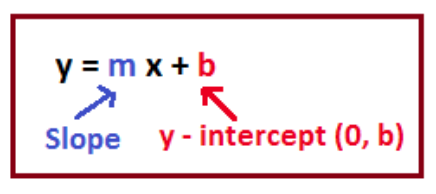# How do you find the slope and intercept of y=-x-7?

Aug 3, 2018

Slope: -1

x-intercept: (-7, 0)

y-intercept: (0, -7)

#### Explanation:

$y = - x - 7$

This equation is in slope-intercept form:Based on the image, we know that the slope is the value multiplied by $x$, so the slope is $- 1$.

We know the $y$-intercept is $b$, or $- 7$, so it is at $\left(0 , - 7\right)$.

To find the $x$-intercept, plug in $0$ for $y$ and solve for $x$:
$0 = - x - 7$

$7 = - x$

$x = - 7$

The $x$-intercept is at $\left(- 7 , 0\right)$.'

Hope this helps!

Aug 4, 2018

Slope $- 1$, $y$-int $- 7$, $x$-int $- 7$

#### Explanation:

The good thing is that this equation is in slope-intercept form

$y = m x + b$, with slope $m$ and a $y$-intercept of $b$.

By pattern matching, we see that our slope is $- 1$, and our $y$-intercept is $- 7$.

We can find our $x$-intercept by setting $y$ equal to zero. We get

$- x - 7 = 0 \implies - x = 7 \implies x = - 7$

Therefore, our slope is $- 1$, our $y$-intercept is $- 7$, and our $x$-intercept is $- 7$.

Hope this helps!